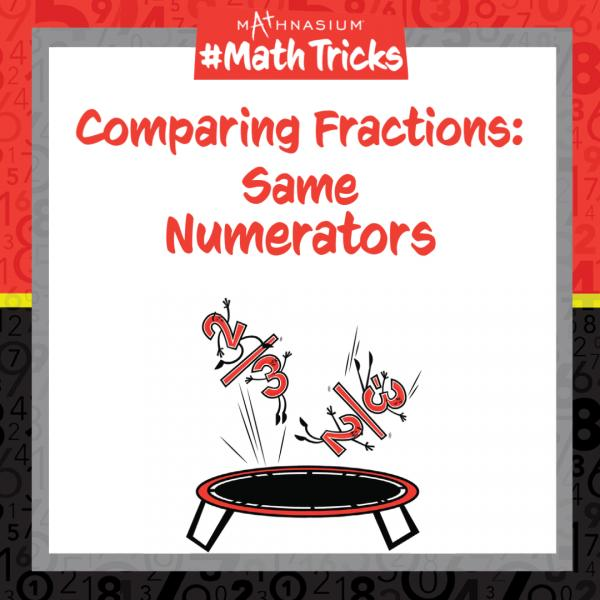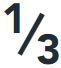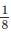# Mathnasium #MathTricks: Fractions (Comparing Fractions Part 2)

By Mathnasium | Added May 11, 2022Welcome to Mathnasium’s Math Tricks series. Today we are comparing fractions that have the same numerators but have different denominators (the total number of equal parts in the whole).

When we compare fractions with the same numerators, we compare the same number of parts; however, the wholes may be divided into a different number of parts. The trick is to compare the denominators.

Recall that, for each whole of the same size:

As the number of equal parts increases, the size of each part becomes smaller.

As the number of equal parts decreases, the size of each part grows bigger.

Follow the example below to identify the fraction with the biggest value.

# Example: You love candy! Would you rather have one piece of a candy bar cut into three equal pieces? Or one piece of the same-size candy bar cut into eight equal pieces?

Step 1: Identify what you are looking for.

You love candy and so you want the biggest piece.

Step 2: Identify the number of pieces you are comparing.

You are comparing one piece from each candy bar.

Step 3: Compare the denominators (the number of pieces each candy bar has

been cut).

Thirds are bigger than eighths.

Answer: One piece of a candy bar cut into three equal pieces, sinceis bigger than.

Now, with this strategy, you are ready to compare fractions with the same numerators.